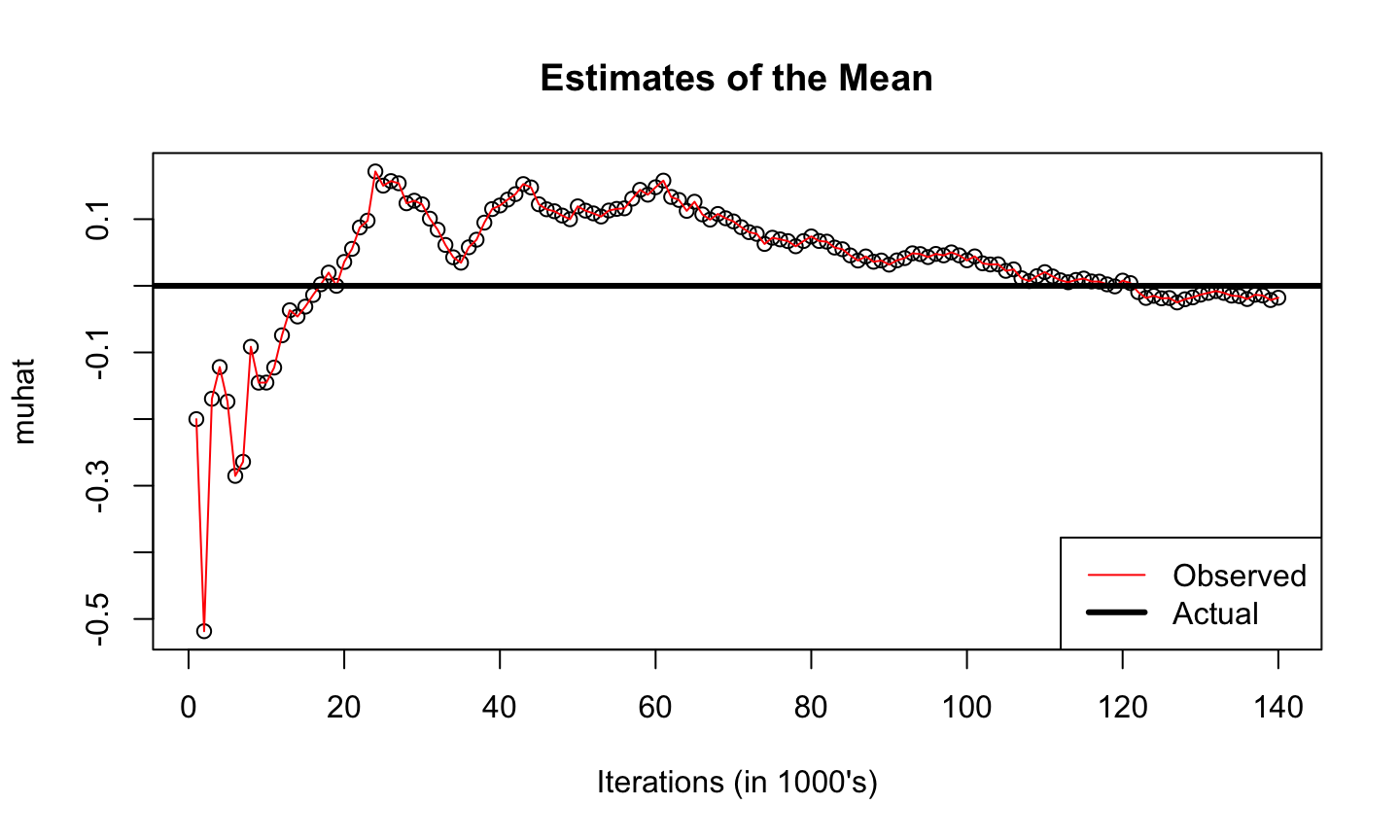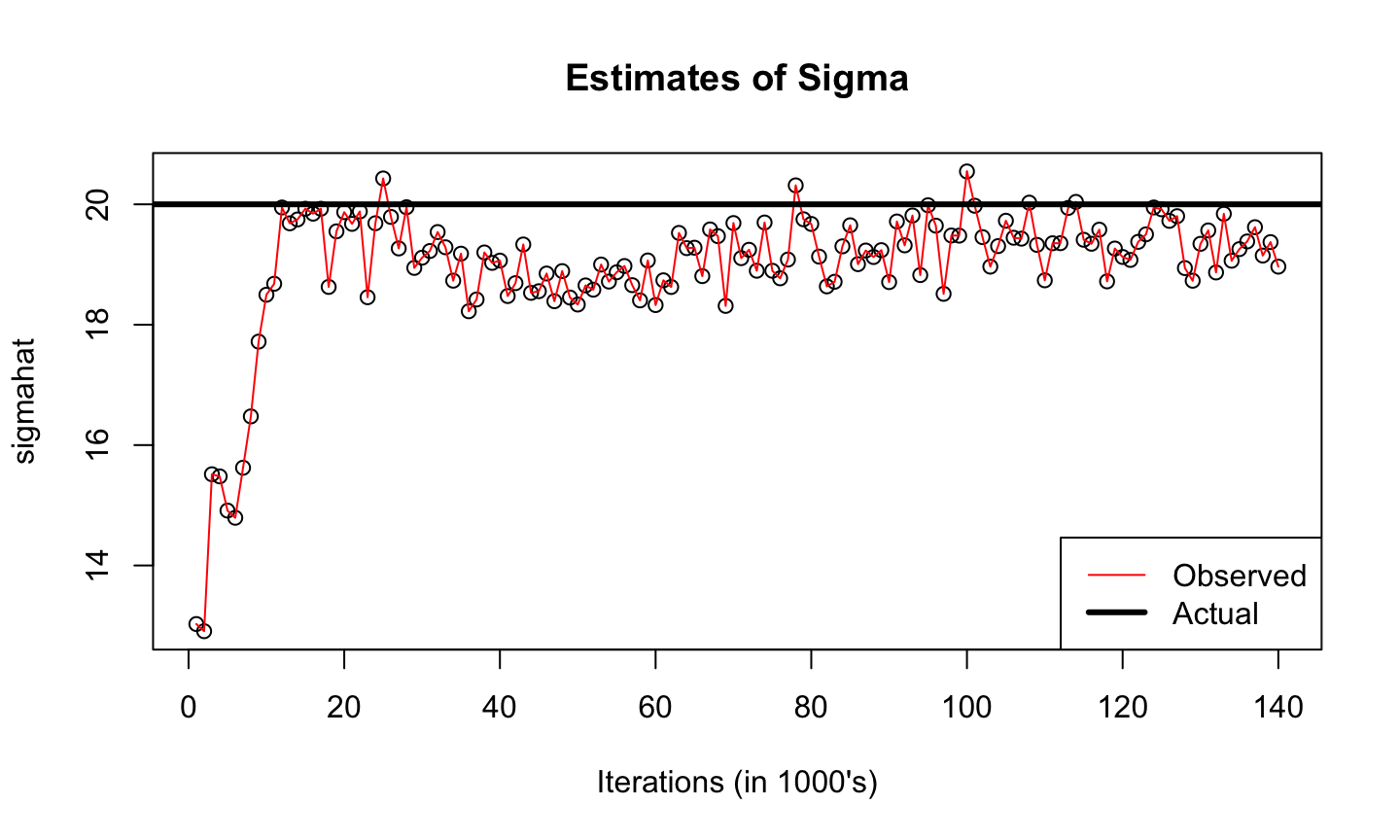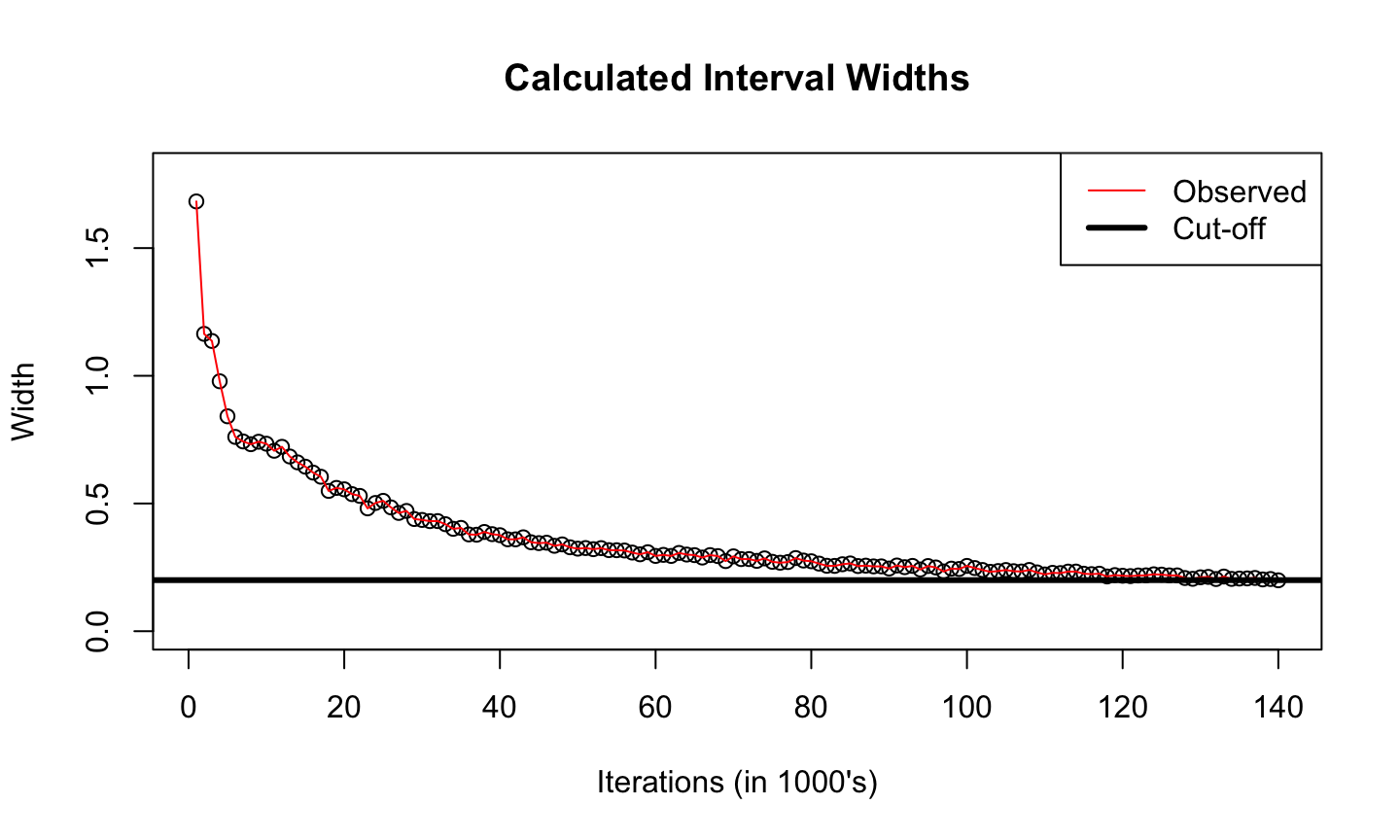## Agenda

• Like Ordinary Monte Carlo (OMC), but better?
• SLLN and Markov chain CLT
• Variance estimation
• AR(1) example
• Metropolis-Hastings algorithm (with an exercise)

## Markov chain Monte Carlo

• A Markov chain is a dependent sequence of random variables $$X_1, X_2, \dots$$ or or random vectors $$X_1, X_2, \dots$$ having the property that the future is independent of the past given the present
• Conditional distribution of $$X_{n+1}$$ given $$X_1, \dots, X_n$$ depends only on $$X_n$$
• The Markov chain has stationary transition probabilities if the conditional distribution of $$X_{n+1}$$ given $$X_n$$ is the same for all $$n$$
• Every Markov chain used in MCMC has this property
• The joint distribution of $$X_1, \dots, X_n$$ is determined by the initial distribution of the Markov chain and the transition probabilities
• Marginal distribution of $$X_1$$
• Conditional distribution of $$X_{n+1}$$ given $$X_n$$

## Markov chain Monte Carlo

• A scalar functional of a Markov chain is a time series, but not necessarily a Markov chain
• A Markov chain is stationary if its initial distribution is stationary
• Different from having stationary transition probabilities
• All chains used in MCMC have stationary transition probabilities, but none are exactly stationary

## Markov chain Monte Carlo

• To be (exactly) stationary, must start the chain with simulation from the equilibrium (invariant, stationary) distribution
• If chain is stationary, then every iterate $$X_i$$ has the same marginal distribution, which is the equilibrium distribution
• If chain is not stationary but has a unique equilibrium distribution, which includes chains used in MCMC, then the marginal distribution $$X_i$$ converges to the equilibrium distribution as $$i \to \infty$$

## Markov chain Monte Carlo

• Let $$\pi$$ be a probability distribution having support $${\cal X} \subseteq \mathbb{R}^{d}$$, $$d \ge 1$$ we want to explore
• When i.i.d. observations are unavailable, a Markov chain with stationary distribution $$\pi$$ can be utilized
• Summarize $$\pi$$ with expectations, quantiles, density plots …

## Markov chain Monte Carlo

• Suppose $$X_1, \dots, X_n$$ are simulation from a Markov chain having a unique equilibrium distribution (say $$\pi$$), and suppose we want to know an expectation $\mu_g = E [ g(X_i) ] = \int_{{\cal X}} g(x) \, \pi(dx)$ where the expectation is with respect to unique equilibrium distribution $$\pi$$
• If $$E_{\pi} |g(X_i)| < \infty$$, then $\hat{\mu}_{n} = \frac{1}{n} \sum_{i=1}^{n} g(X_i) \stackrel{a.s.}{\rightarrow} \mu_g \quad \text{as } n \rightarrow \infty \; (SLLN).$

## Markov chain Monte Carlo

• The central limit theorem (CLT) for Markov chains says $\sqrt{n} (\hat{\mu}_{n} - E_{\pi} g (X_i)) \to \text{N} (0, \sigma^{2}) \; ,$ where $\sigma^{2} = \text{Var} g(X_i) + 2 \sum_{k = 1}^{\infty} \text{Cov} \left[ g(X_i), g(X_{i+k})\right]$
• CLT holds if $$E_{\pi} |g (X_i) |^{2+\epsilon} < \infty$$ and the Markov chain is geometrically ergodic
• Can estimate $$\sigma^{2}$$ in various ways
• Verifying such a mixing condition is generally very challenging
• Nevertheless, we expect the CLT to hold in practice when using a smart sampler

## Batch means

• In order to make MCMC practical, need a method to estimate the variance $$\sigma^{2}$$ in the CLT, then can proceed just like in OMC
• If $$\hat{\sigma}^{2}$$ is a consistent estimate of $$\sigma^{2}$$, then an asymptotic 95% confidence interval for $$\mu_g$$ is $\hat{\mu}_{n} \pm 1.96 \frac{\hat{\sigma}}{\sqrt{n}}$
• The method of batch means estimates the asymptotic variance for a stationary time series

## Batch means

• Markov chain CLT says $\hat{\mu}_{n} \approx \text{N} \left( \mu_g, \frac{\sigma^{2}}{n} \right)$
• Suppose $$b$$ evenly divides $$n$$ and we have the means $\hat{\mu}_{b,k} = \frac{1}{b} \sum_{i=bk+1}^{bk+b} g(X_i)$ for $$k = 1, \dots, a = n / b$$
• Then each of these batch means satisfies (if $$b$$ is sufficiently large) $\hat{\mu}_{b,k} \approx \text{N} \left( \mu_g, \frac{\sigma^{2}}{b} \right)$

## Batch means

• Thus empirical variance of the sequence of batch means $\frac{1}{a} \sum_{k=1}^a \left( \hat{\mu}_{b,k} - \hat{\mu}_{n} \right)^2$ estimates $$\sigma^{2}/b$$
• And $$b/n$$ times this estimates $$\sigma^{2} / n$$, the asymptotic variance of $$\hat{\mu}_{n}$$
• Batch means can produce a strongly consistent estimator of $$\sigma^{2}$$ if $$b \rightarrow \infty$$ and $$a \rightarrow \infty$$ as $$n \rightarrow \infty$$

## Stopping rules

• Suppose $$\epsilon>0$$, then a **fixed-width stopping rule* terminates the simulation the first time half-width (or width) of a confidence interval is sufficiently small
• That is, simulate until $1.96 \frac{\hat{\sigma}}{\sqrt{n}} < \epsilon .$

## Example: AR(1)

• Consider the Markov chain such that $X_i = \rho X_{i-1} + \epsilon_i$ where $$\epsilon_i \stackrel{iid}{\sim} N(0,1)$$
• Consider $$X_1 = 0$$, $$\rho = .95$$, and estimating $$E_{\pi} X = 0$$
• Run until $w_{n} = 2 z_{.975} \frac{ \hat{\sigma}}{ \sqrt{n} } \leq 0.2$ where $$\hat{\sigma}$$ is calculated using batch means

## Example: AR(1)

The following will provide an observation from the MC 1 step ahead

ar1 <- function(m, rho, tau) {
rho*m + rnorm(1, 0, tau)
}

Next, we add to this function so that we can give it a Markov chain and the result will be p observations from the Markov chain

ar1.gen <- function(mc, p, rho, tau, q=1) {
loc <- length(mc)
junk <- double(p)
mc <- append(mc, junk)

for(i in 1:p){
j <- i+loc-1
mc[(j+1)] <- ar1(mc[j], rho, tau)
}
return(mc)
}

## Example: AR(1)

set.seed(20)
library(mcmcse)
## mcmcse: Monte Carlo Standard Errors for MCMC
## Version 1.2-1 created on 2016-03-24.
## copyright (c) 2012, James M. Flegal, University of California,Riverside
##                     John Hughes, University of Minnesota
##                     Dootika Vats, University of Minnesota
##  For citation information, type citation("mcmcse").
##  Type help("mcmcse-package") to get started.
tau <- 1
rho <- .95
out <- 0
eps <- 0.1
start <- 1000
r <- 1000

## Example: AR(1)

out <- ar1.gen(out, start, rho, tau)
MCSE <- mcse(out)$se N <- length(out) t <- qt(.975, (floor(sqrt(N) - 1))) muhat <- mean(out) check <- MCSE * t while(eps < check) { out <- ar1.gen(out, r, rho, tau) MCSE <- append(MCSE, mcse(out)$se)
N <- length(out)
t <- qt(.975, (floor(sqrt(N) - 1)))
muhat <- append(muhat, mean(out))
check <- MCSE[length(MCSE)] * t
}

N <- seq(start, length(out), r)
t <- qt(.975, (floor(sqrt(N) - 1)))
half <- MCSE * t
sigmahat <- MCSE*sqrt(N)
N <- seq(start, length(out), r) / 1000

## Example: AR(1)

plot(N, muhat, main="Estimates of the Mean", xlab="Iterations (in 1000's)")
points(N, muhat, type="l", col="red") ; abline(h=0, lwd=3)
legend("bottomright", legend=c("Observed", "Actual"), lty=c(1,1), col=c(2,1), lwd=c(1,3))## Example: AR(1)

plot(N, sigmahat, main="Estimates of Sigma", xlab="Iterations (in 1000's)")
points(N, sigmahat, type="l", col="red"); abline(h=20, lwd=3)
legend("bottomright", legend=c("Observed", "Actual"), lty=c(1,1), col=c(2,1), lwd=c(1,3))## Example: AR(1)

plot(N, 2*half, main="Calculated Interval Widths", xlab="Iterations (in 1000's)", ylab="Width", ylim=c(0, 1.8))
points(N, 2*half, type="l", col="red"); abline(h=0.2, lwd=3)
legend("topright", legend=c("Observed", "Cut-off"), lty=c(1,1), col=c(2,1), lwd=c(1,3))## Markov chain Monte Carlo

• MCMC methods are used most often in Bayesian inference where the equilibrium (invariant, stationary) distribution is a posterior distribution
• Challenge lies in construction of a suitable Markov chain with $$f$$ as its stationary distribution
• A key problem is we only get to observe $$t$$ observations from $$\left\{ X_t \right\}$$, which are serially dependent

• Other questions to consider
• How good are my MCMC estimators?
• How long to run my Markov chain simulation?
• How to compare MCMC samplers?
• What to do in high-dimensional settings?

## Metropolis-Hastings algorithm

Setting $$X_0 = x_0$$ (somehow), the Metropolis-Hastings algorithm generates $$X_{t+1}$$ given $$X_t = x_t$$ as follows:

1. Sample a candidate value $$X^* \sim g(\cdot | x_t)$$ where $$g$$ is the proposal distribution
2. Compute the MH ratio $$R(x_t, X^*)$$, where $R(x_t, X^*) = \frac{f(x^*) g (x_t | x^*)}{f(x_t) g (x^* | x_t)}$
3. Set $X_{t+1} = \begin{cases} x^* \mbox{ w.p.\ } \min\{ R(x_t, X^*), 1\} \\ x_t \mbox{ otherwise} \end{cases}$

## Metropolis-Hastings algorithm

• Irreducibility and aperiodicity depend on the choice of $$g$$, these must be checked
• Performance (finite sample) depends on the choice of $$g$$ also, be careful

## Independence chains

• Suppose $$g (x^* | x_t) = g (x^*)$$, this yields an independence chain since the proposal does not depend on the current state
• In this case, the MH ratio is
$R(x_t, X^*) = \frac{f(x^*) g (x_t)}{f(x_t) g (x^*)},$ and the resulting Markov chain will be irreducible and aperiodic if $$g > 0$$ where $$f>0$$

• A good envelope function $$g$$ should resemble $$f$$, but should cover $$f$$ in the tails

## Random walk chains

• Generate $$X^*$$ such that $$\epsilon\sim h(\cdot)$$ and set $$X^* = X_t + \epsilon$$, then $$g(x^* | x_t) = h(x^* - x_t)$$
• Common choices of $$h(\cdot)$$ are symmetric zero mean random variables with a scale parameter, e.g. a Uniform($$-a,a$$), Normal($$0, \sigma^2$$), $$c*T_{\nu}, \dots$$

• For symmetric zero mean random variables, the MH ratio is
$R(x_t, X^*) = \frac{f(x^*)}{f(x_t)}$
• If the support of $$f$$ is connected and $$h$$ is positive in a neighborhood of 0, then the chain is irreducible and aperiodic.

## Example: Markov chain basics

Exercise: Suppose $$f \sim Exp(1)$$

1. Write an independence MH sampler with $$g \sim Exp(\theta)$$
2. Show $$R(x_t, X^*) = \exp \left\{ (x_t - x^*)(1-\theta) \right\}$$
3. Generate 1000 draws from $$f$$ with $$\theta \in \{ 1/2, 1, 2 \}$$
4. Write a random walk MH sampler with $$h \sim N(0, \sigma^2)$$
5. Show $$R(x_t, X^*) = \exp \left\{ x_t - x^* \right \} I(x^* > 0)$$
6. Generate 1000 draws from $$f$$ with $$\sigma \in \{ .2, 1, 5 \}$$
7. In general, do you prefer an independence chain or a random walk MH sampler? Why?
8. Implement the fixed-width stopping rule for you preferred chain

## Example: Markov chain basics

Independence Metropolis sampler with Exp($$\theta$$) proposal

ind.chain <- function(x, n, theta = 1) {
## if theta = 1, then this is an iid sampler
m <- length(x)
x <- append(x, double(n))
for(i in (m+1):length(x)){
x.prime <- rexp(1, rate=theta)
u <- exp((x[(i-1)]-x.prime)*(1-theta))
if(runif(1) < u)
x[i] <- x.prime
else
x[i] <- x[(i-1)]
}
return(x)
}

## Example: Markov chain basics

Random Walk Metropolis sampler with N($$0,\sigma$$) proposal

rw.chain <- function(x, n, sigma = 1) {
m <- length(x)
x <- append(x, double(n))
for(i in (m+1):length(x)){
x.prime <- x[(i-1)] + rnorm(1, sd = sigma)
u <- exp((x[(i-1)]-x.prime))
u
if(runif(1) < u && x.prime > 0)
x[i] <- x.prime
else
x[i] <- x[(i-1)]
}
return(x)
}

## Example: Markov chain basics

trial0 <- ind.chain(1, 500, 1)
trial1 <- ind.chain(1, 500, 2)
trial2 <- ind.chain(1, 500, 1/2)
rw1 <- rw.chain(1, 500, .2)
rw2 <- rw.chain(1, 500, 1)
rw3 <- rw.chain(1, 500, 5)